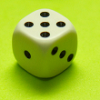You may also likeThe Lady or the Lions

The King showed the Princess a map of the maze and the Princess was allowed to decide which room she would wait in. She was not allowed to send a copy to her lover who would have to guess which path to follow. Which room should she wait in to give her lover the greatest chance of finding her?Four fair dice are marked differently on their six faces. Choose first ANY one of them. I can always choose another that will give me a better chance of winning. Investigate.Nine or Ten?

Is a score of 9 more likely than a score of 10 when you roll three dice?

Strange Dice

Age 11 to 14 Short Challenge Level:

Listing the possibilities
The 1 on the first die could come up with any of the numbers on the second die, which gives 6 options - 2, 3, 4, 6, 8, 10. We can record them in a table, as shown below. We can put some of them in twice, to show getting a 1 on the second die instead - so for example, we could get a 3 from a 1 on the first die and a 2 on the second, a 2 on the first die and a 1 on the second.
2 3 4 6 8 10
1+1 1+2
2+1
1+3
3+1
1+5
5+1
1+7
7+1
1+9
9+1

The 2 on the first die could also come up with any of the numbers on the second die, which gives 3, 4, 5, 7, 9, 11. However, 3 and 4 have already been counted, so we should just add 5, 7, 9 and 11 to the list of possibilities: 2, 3, 4, 5, 6, 7, 8, 9, 10, 11. To determine later which sums are most likely, we should still add the sums involving 2s to their columns.
2 3 4 5 6 7 8 9 10 11
1+1 1+2
2+1
1+3
3+1
2+2
2+3
3+2
1+5
5+1
2+5
5+2
1+7
7+1
2+7
7+2
1+9
9+1
2+9
9+2

For the 3, we only need to add numbers greater than 11 to the list of possibilities - so 3 + 9 = 12, which gives 2, 3, 4, 5, 6, 7, 8, 9, 10, 11, 12. To later see which possibilities are most likely, we should write in all of the options for the 3s - starting from 3 + 3 = 6, since 3 with 1 and 2 have already been counted.
2 3 4 5 6 7 8 9 10 11 12
1+1 1+2
2+1
1+3
3+1
2+2
2+3
3+2
1+5
5+1
3+3
2+5
5+2
1+7
7+1
3+5
5+3
2+7
7+2
1+9
9+1
3+7
7+3
2+9
9+2
3+9
9+3

The same is true for the 5 - we can't use the 5 to make 13, but 5 + 9 = 14, so we have 2, 3, 4, 5, 6, 7, 8, 9, 10, 11, 12, 14. We can also make 10 and 12 again.
2 3 4 5 6 7 8 9 10 11 12 14
1+1 1+2
2+1
1+3
3+1
2+2
2+3
3+2
1+5
5+1
3+3
2+5
5+2
1+7
7+1
3+5
5+3
2+7
7+2
1+9
9+1
3+7
7+3
5+5
2+9
9+2
3+9
9+3
5+7
7+5
5+9
9+5

For the 7, 7 + 7 = 14 has already been counted, and we know we can't use the 7 with a smaller number to fill in the gap at 13 (or we would have filled the gaps when we considered the smaller number). So all that is left is 7 + 9 = 16, and 9 + 9 = 18.
2 3 4 5 6 7 8 9 10 11 12 14 16 18
1+1 1+2
2+1
1+3
3+1
2+2
2+3
3+2
1+5
5+1
3+3
2+5
5+2
1+7
7+1
3+5
5+3
2+7
7+2
1+9
9+1
3+7
7+3
5+5
2+9
9+2
3+9
9+3
5+7
7+5
5+9
9+5
7+7
7+9
9+7
9+9

There are 14 different possible totals: 2, 3, 4, 5, 6, 7, 8, 9, 10, 11, 12, 14, 16, 18.

The sum that is most likely to come up is 10, because there are more different ways to make 10 than any other number.

Using an addition table
The following table shows all of the possible sums:
First die
Second die + 1 2 3 5 7 9
1 2 3 4 6 8 10
2 3 4 5 7 9 11
3 4 5 6 8 10 12
5 6 7 8 10 12 14
7 8 9 10 12 14 16
9 10 11 12 14 16 18

So the possible sums are: 2, 3, 4, 5, 6, 7, 8, 9, 10, 11, 12, 14, 16, 18.

For each die, each of the numbers is equally likely to come up. So the sum is equally likely to take the value of any of the cells in the table - so each cell represents a probability of $\frac1{36}$ (since there are 6$\times$6 = 36 cells altogether).

10 appears the most times - 5 times - so it is the most likely sum. The probability of getting 10 is $\frac5{36}$.
You can find more short problems, arranged by curriculum topic, in our short problems collection.